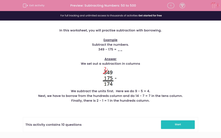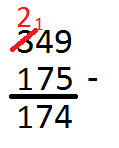# Subtracting Numbers: 50 to 500

In this worksheet, students practice formal written methods of subtraction for sums involving two three-digit numbers and one three-digit and one two-digit number.This content is premium and exclusive to EdPlace subscribers.Key stage:  KS 2

Curriculum topic:   Maths and Numerical Reasoning

Curriculum subtopic:   Mixed Problems

Difficulty level:#### Worksheet Overview

In this worksheet, you will practise subtraction with borrowing.

Example

Subtract the numbers.

349 - 175 = __

We set out a subtraction in columnsWe subtract the units first.  Here we do 9 - 5 = 4.

Next, we have to borrow from the hundreds column and do 14 - 7 = 7 in the tens column.

Finally, there is 2 - 1 = 1 in the hundreds column.

### What is EdPlace?

We're your National Curriculum aligned online education content provider helping each child succeed in English, maths and science from year 1 to GCSE. With an EdPlace account you’ll be able to track and measure progress, helping each child achieve their best. We build confidence and attainment by personalising each child’s learning at a level that suits them.

Get started